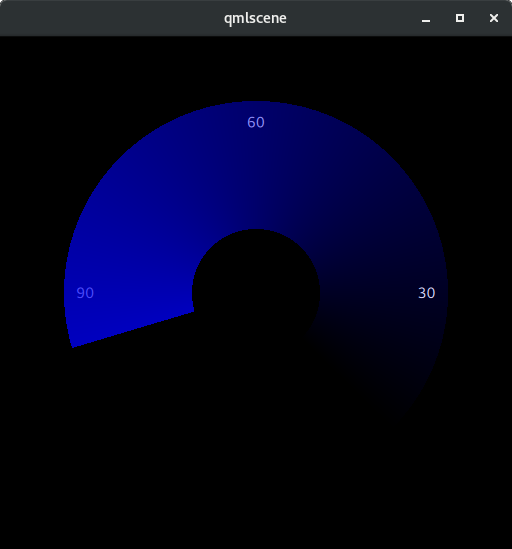# OpenGL fragmentShader to draw anti-clockwise

• I am working with QML for some time but new to OpenGL. I was trying to understand how the fragmentShader is working in the reference reply. I tried to use he code to move the needle anti-clockwise. In that case the shader effect will be also anti clockwise, so I started from +145 deg instead of - 45 deg in the reference.

``````fragmentShader: "
uniform lowp float qt_Opacity;
uniform highp float angleBase;
uniform highp float angle;
varying highp vec2 coord;
void main() {
gl_FragColor = vec4(0.0,0.0,0.0,0.0);
highp vec2 d=2.0*coord-vec2(1.0,1.0);
highp float r=length(d);
if (0.25<=r && r<=0.75) {
highp float a=atan2(d.x,-d.y);
if (angleBase<=a && a<=angle) {
highp float p=(a-angleBase)/(angle-angleBase);
gl_FragColor = vec4(0.0,0.0,p,p) * qt_Opacity;
}
}
}"
``````

The value of 'a' obtained from the 'atan2' function determines the angle 'theta' and draws withing the 'r' value of 0.25 a,d 0.75 with selected color, creating a background shader effect as the needle moves. But If I want to move the needle anticlockwise, as far as I understand the angle will be (pi - a) and baseAngle will be +2.53073 instead if -2.53073. But this doesn't work. Can someone help me to understand this code and how do I paint the other side of the needle (needle moving anti clockwise).

• Hi. Here's the original clockwise dial from that linked post. Still works in 5.15's qmlscene.

``````import QtQuick 2.2

Rectangle {
id: main
width: 512
height: 512
color: 'black'

// Put some text in the background just to check opacity
Text {
x: main.width/6.0-0.5*contentWidth
y: main.height/2.0-0.5*contentHeight
text: '30'
color: 'white'
}
Text {
x: main.width/2.0-0.5*contentWidth
y: main.height/6.0-0.5*contentHeight
text: '60'
color: 'white'
}
Text {
x: 5.0*main.width/6.0-0.5*contentWidth
y: main.height/2.0-0.5*contentHeight
text: '90'
color: 'white'
}

id: gauge
anchors.fill: parent

opacity: 0.75  // Making it totally opaque on leading edge obscures the number!

// Angles measured clockwise from up, in range -pi to pi
property real angleBase: -pi*0.75
property real angle

uniform highp mat4 qt_Matrix;
attribute highp vec4 qt_Vertex;
attribute highp vec2 qt_MultiTexCoord0;
varying highp vec2 coord;
void main() {
coord = qt_MultiTexCoord0;
gl_Position = qt_Matrix * qt_Vertex;
}"

uniform lowp float qt_Opacity;
uniform highp float angleBase;
uniform highp float angle;
varying highp vec2 coord;
void main() {
gl_FragColor = vec4(0.0,0.0,0.0,0.0);
highp vec2 d=2.0*coord-vec2(1.0,1.0);
highp float r=length(d);
if (0.25<=r && r<=0.75) {
highp float a=atan(d.x,-d.y);
if (angleBase<=a && a<=angle) {
highp float p=(a-angleBase)/(angle-angleBase);
gl_FragColor = vec4(0.0,0.0,p,p) * qt_Opacity;
}
}
}"
}

// Animate the gauge position
SequentialAnimation {
running: true
loops: Animation.Infinite
NumberAnimation {
from: gauge.angleBase
to: gauge.angleBase+1.5*gauge.pi
duration: 1000
target: gauge
property: 'angle'
easing.type: Easing.InOutSine
}
NumberAnimation {
from: gauge.angleBase+1.5*gauge.pi
to: gauge.angleBase
duration: 1000
target: gauge
property: 'angle'
easing.type: Easing.InOutSine
}
}
}
``````

And here's a version which is the mirror image. It measures angles in the same sense (positive=clockwise, with zero "up"), but the "base" for the dial has moved over to the positive domain and the dial angle dials back from that:

``````import QtQuick 2.2

Rectangle {
id: main
width: 512
height: 512
color: 'black'

// Put some text in the background just to check opacity
Text {
x: main.width/6.0-0.5*contentWidth
y: main.height/2.0-0.5*contentHeight
text: '90'
color: 'white'
}
Text {
x: main.width/2.0-0.5*contentWidth
y: main.height/6.0-0.5*contentHeight
text: '60'
color: 'white'
}
Text {
x: 5.0*main.width/6.0-0.5*contentWidth
y: main.height/2.0-0.5*contentHeight
text: '30'
color: 'white'
}

id: gauge
anchors.fill: parent

opacity: 0.75  // Making it totally opaque on leading edge obscures the number!

// Angles measured clockwise from up, in range -pi to pi
property real angleBase: pi*0.75  // Move dial baseline over to the right as we are going to dial backwards, anti-clockwise from here
property real angle

uniform highp mat4 qt_Matrix;
attribute highp vec4 qt_Vertex;
attribute highp vec2 qt_MultiTexCoord0;
varying highp vec2 coord;
void main() {
coord = qt_MultiTexCoord0;
gl_Position = qt_Matrix * qt_Vertex;
}"

uniform lowp float qt_Opacity;
uniform highp float angleBase;
uniform highp float angle;
varying highp vec2 coord;
void main() {
gl_FragColor = vec4(0.0,0.0,0.0,0.0);
highp vec2 d=2.0*coord-vec2(1.0,1.0);
highp float r=length(d);
if (0.25<=r && r<=0.75) {
highp float a=atan(d.x,-d.y);
if (angle<=a && a<=angleBase) {   // Shaded domain changes to be below dial baseline
highp float p=(a-angleBase)/(angle-angleBase);
gl_FragColor = vec4(0.0,0.0,p,p) * qt_Opacity;
}
}
}"
}

// Animate the gauge position
SequentialAnimation {
running: true
loops: Animation.Infinite
NumberAnimation {
from: gauge.angleBase
to: gauge.angleBase-1.5*gauge.pi  // We will be rotating back anti-clockwise
duration: 1000
target: gauge
property: 'angle'
easing.type: Easing.InOutSine
}
NumberAnimation {
from: gauge.angleBase-1.5*gauge.pi  // We will be rotating back anti-clockwise
to: gauge.angleBase
duration: 1000
target: gauge
property: 'angle'
easing.type: Easing.InOutSine
}
}
}
``````

Looks like this:To make it clear how I've adapted it, here's the diffs between the two files:

``````\$ diff dial.qml dial-ccw.qml
13c13
<     text: '30'
---
>     text: '90'
25c25
<     text: '90'
---
>     text: '30'
37c37
<     property real angleBase: -pi*0.75
---
>     property real angleBase: pi*0.75  // Move dial baseline over to the right as we are going to dial backwards, anti-clockwise from here
63c63
<           if (angleBase<=a && a<=angle) {
---
>           if (angle<=a && a<=angleBase) {   // Shaded domain changes to be below dial baseline
77c77
<       to: gauge.angleBase+1.5*gauge.pi
---
>       to: gauge.angleBase-1.5*gauge.pi  // We will be rotating back anti-clockwise
84c84
<       from: gauge.angleBase+1.5*gauge.pi
---
>       from: gauge.angleBase-1.5*gauge.pi  // We will be rotating back anti-clockwise

``````

As a general principle: if I have something working on the basis of one particular coordinate system (in this case, the idea that angles are measures clockwise from zero at vertical up), then I generally find changes are easier continuing to work in that coordinate system and expressing the new behaviour in it, rather than trying to establish a new coordinate system. I find that works best for me anyway!

Obviously for a more real implementation a dial component would probably expose some generic 0-1 property controlling the position (`property real value`, say) and the angle property would be an internal detail bound to `angleBase+value*1.5*pi` or `angleBase-value*1.5*pi` respectively in the clockwise and counter-clockwise versions.